International
Tables for
Crystallography
Volume D
Physical properties of crystals
Edited by A. Authier

International Tables for Crystallography (2006). Vol. D, ch. 1.1, pp. 22-24

## Section 1.1.4.9.9. Symmetric tensors of rank 4

A. Authiera*

aInstitut de Minéralogie et de la Physique des Milieux Condensés, Bâtiment 7, 140 rue de Lourmel, 75015 Paris, France
Correspondence e-mail: aauthier@wanadoo.fr

#### 1.1.4.9.9. Symmetric tensors of rank 4

| top | pdf |

For symmetric tensors such as those representing principal properties, one finds the following, representing the nonzero components for the leading diagonal and for one half of the others.

#### 1.1.4.9.9.1. Triclinic system

| top | pdf |There are 45 independent coefficients.

#### 1.1.4.9.9.2. Monoclinic system

| top | pdf |There are 25 independent coefficients.

#### 1.1.4.9.9.3. Orthorhombic system

| top | pdf |There are 15 independent coefficients.

#### 1.1.4.9.9.4. Trigonal system

| top | pdf |

 (i) Groupsandwith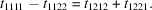There are 15 independent components. (ii) Groups,,withThere are 11 independent components.

#### 1.1.4.9.9.5. Tetragonal system

| top | pdf |

 (i) Groups,,There are 13 independent components. (ii) Groups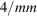,,,There are 9 independent components.

#### 1.1.4.9.9.6. Hexagonal and cylindrical systems

| top | pdf |

 (i) Groups,,;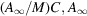withThere are 12 independent components. (ii) Groups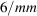,,,;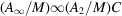,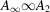withThere are 10 independent components.

#### 1.1.4.9.9.7. Cubic system

| top | pdf |

 (i) Groups,withThere are 5 independent components. (ii) Groups,,, and spherical system: the reduced tensors are already symmetric (see Sections 1.1.4.9.7and 1.1.4.9.8).